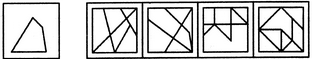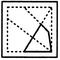# Non Verbal Reasoning - Embedded Images - Discussion

### Discussion :: Embedded Images - Section 1 (Q.No.17)

In each of the following questions, you are given a figure (X) followed by four alternative figures (1), (2), (3) and (4) such that figure (X) is embedded in one of them. Trace out the alternative figure which contains fig. (X) as its part.

17.

Find out the alternative figure which contains figure (X) as its part.(X)                (1)         (2)         (3)        (4)

 [A]. 1 [B]. 2 [C]. 3 [D]. 4

Explanation:Ankur Kumar said: (Jul 3, 2020) I think A is the correct answer.

 A.Sai Pramod said: (Oct 23, 2020) Both A and B are correct. Anyone, please explain.

 Himanshu said: (Feb 20, 2021) Yes, A can be the answer.

 Mayank said: (Jun 12, 2021) I think A is correct because B is small as compare to real image.

 Niranjana said: (Sep 11, 2021) B is the correct answer even though it is small, A isn't because the right side is straight in A which isn't the same as the question.

 Niranjana said: (Sep 11, 2021) B is the correct answer even though it is small, A isn't because the right side is straight in A which isn't the same as the question.